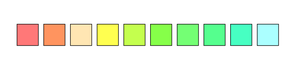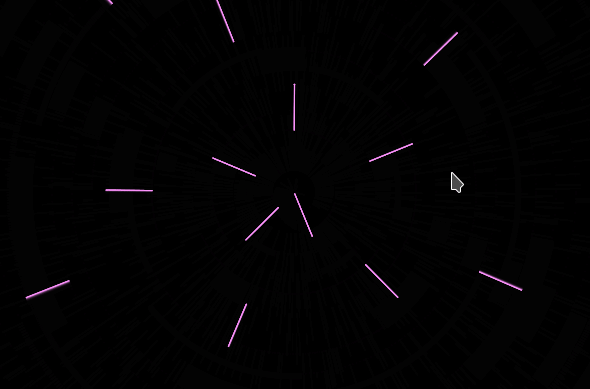# p5.js colorMode() Function

• Last Updated : 31 Oct, 2021

The colorMode() function is an inbuilt function in p5.js which is used to let the user choose between RGB or HSB color options. The RGB color mode is by default. Thus, the parameters that which the user passes into it corresponds to red, green and blue values. The user creates various colors by passing in numbers (parameters which ranger between 0 to 255) for these values, therefore, the resulting color is a blend of red, green, and blue. There is another color mode called HSB that uses hue, saturation and brightness values for defining the color.

Syntax:

```colorMode(mode, [value])
colorMode(mode, value1, value2, value3, [valueA])```

Parameters:

• mode: It is the mode of the color that has to bet set. This can be set to RGB or HSB. It is the equivalent to Red/Green/Blue or Hue/Saturation/Brightness.
• value: It is a number that denotes the range for all values between 0 to 255. It is an optional value.
• value1: It is a number that denotes the range for the red or hue value.
• value2: It is a number that denotes the range for the green or saturation value depending on the current color mode.
• value3: It is a number that denotes the range for the range for the blue or brightness/lightness values depending on the current color mode
• valueA: It is a number that denotes the range for the alpha value, It is between 0 and 255. It is an optional value.

Below programs illustrates the colorMode() function in p5.js:

Example 1:

## Javascript

 `function` `setup() { ``    ``createCanvas(600, 600);``    ``colorMode(HSB, 360, 100, 100);``    ``noLoop();``}`` ` `function` `draw() { ``    ``background(0, 0, 100);``   ` `    ``for` `(``var` `i = 0; i < 10; i = i + 1) {``        ``var` `x = 50 + i * 50;``        ``var` `y = 300;``        ``var` `h = i * 20;``        ``var` `s = random(20, 80);``        ``fill(h, s, 100);``        ``rect (x, y, 40, 40);``    ``}``}`

Output:Example 2:

## Javascript

 `function` `setup() {``    ``createCanvas(600, 400);``    ``background(0);``    ``colorMode(RGB, 78);``}`` ` `function` `draw() {``    ``fill(0, 0, 0, 10);``    ``rect(-1, -1, 1401, 901);``    ``stroke(2 * frameCount, mouseX/10, 255);``    ``translate(width/2, height/2);``    ``for` `(let i = 2; i < 400; i = i+20) {``        ``rotate(``            ``noise(frameCount * 0.0004) * (1000/mouseX)``        ``);``        ``line(i, 0, ((mouseX/10) + i), 0);``    ``}``}`

Output:My Personal Notes arrow_drop_up